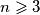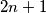### MEMO 2017 pojedinačno problem 2

Kvaliteta:
Avg: 0,0
Težina:
Avg: 6,0

Let$n \geqslant 3$ be an integer. A labelling of the$n$ vertices, the$n$ sides and the interior of a regular$n$-gon by$2n + 1$ distinct integers is called memorable if the following conditions hold:

(a) Each side has a label that is the arithmetic mean of the labels of its endpoints.
(b) The interior of the$n$-gon has a label that is the arithmetic mean of the labels of all the vertices.

Determine all integers$n \geqslant 3$ for which there exists a memorable labelling of a regular$n$-gon consisting of$2n + 1$ consecutive integers.

Izvor: Srednjoeuropska matematička olimpijada 2017, pojedinačno natjecanje, problem 2